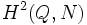# Supergroups of cyclic group:Z4

## Contents

View supergroups of particular groups | View other specific information about cyclic group:Z4

This article discusses some of the supergroups of the cyclic group of order four.

## Classification of supergroups of order eight

Further information: Classification of finite p-groups with cyclic maximal subgroup

### The general procedure

We classify groups$G$ of order eight containing the cyclic group of order four as a normal subgroup$N$. The quotient group$G/N$ must therefore be isomorphic to the cyclic group of order two.

The general procedure is as follows:

• Determine all homomorphisms from$Q$ to$\operatorname{Aut}(N)$.
• For each of these automorphisms, find all possible extensions, classified by the second cohomology group$H^2(Q,N)$ for that action.

### The classification$\operatorname{Aut}(N)$ is cyclic of order two, so there are two possible homomorphisms from$Q$ to$\operatorname{Aut}(N)$: the trivial homomorphism and the unique isomorphism.

The groups corresponding to the trivial homomorphism are:

• Direct product of Z4 and Z2: This corresponds to the identity element in$H^2(Q,N)$.
• Cyclic group:Z8: This corresponds to the non-identity element in$H^2(Q,N)$.

The groups corresponding to the unique isomorphism are:

• Dihedral group:D8: This corresponds to the identity element in$H^2(Q,N)$.
• Quaternion group: This corresponds to the non-identity element in$H^2(Q,N)$.